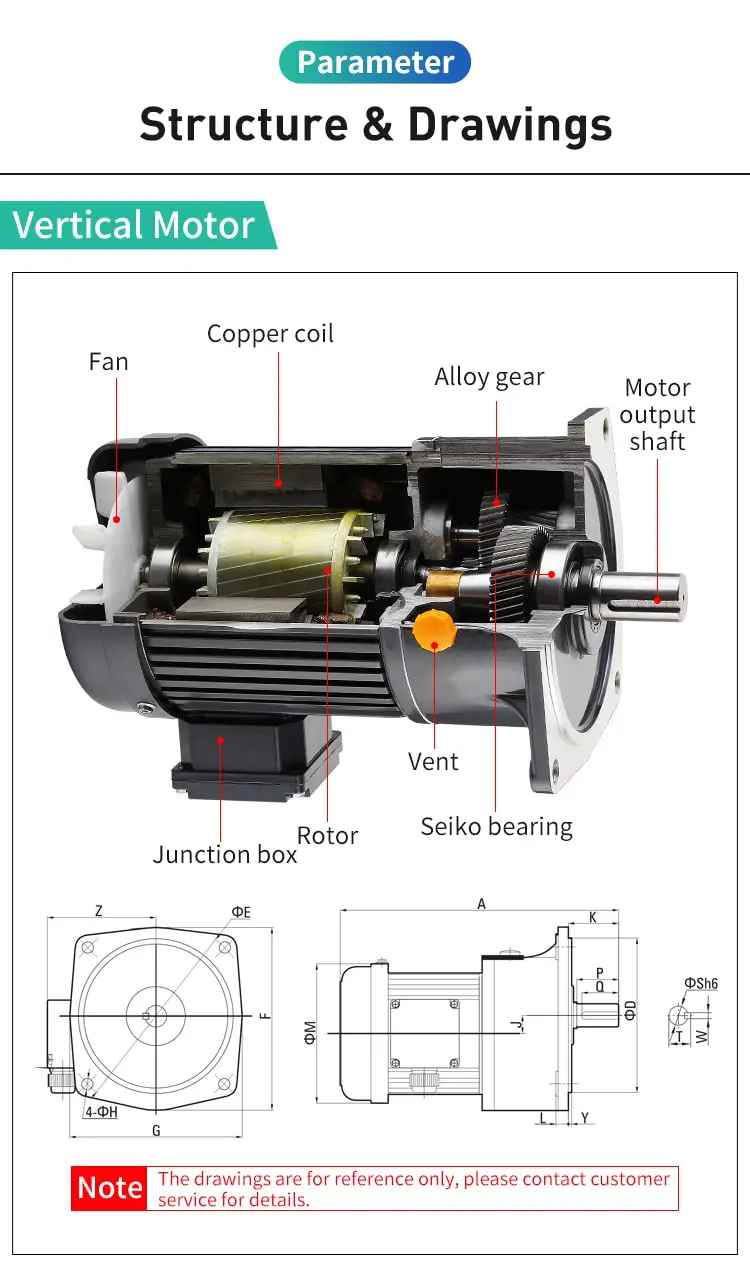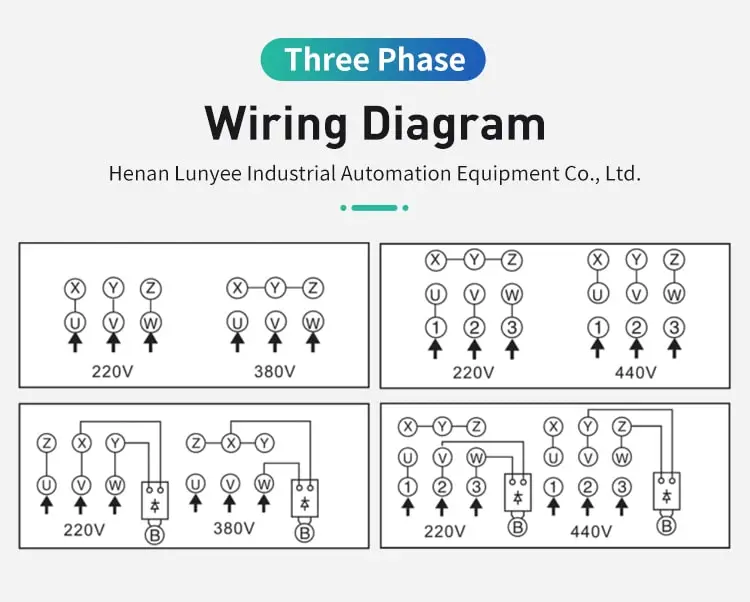By Jasmine 2023-07-28 09:05:30

#### What is synchronous speed in induction motor?

Synchronous speed is the speed at which the rotating magnetic field in the stator of an induction motor rotates. It is determined by the frequency of the power supply and the number of poles in the motor.

Synchronous speed is a term used in the context of induction motors to refer to the speed at which the magnetic field of the stator rotates in relation to the rotor. In an induction motor, the magnetic field of the stator drives the rotor, causing it to rotate and generate rotational motion. The speed of the stator's magnetic field is known as the synchronous speed, and it is an important parameter in the operation of an induction motor.

The synchronous speed of an induction motor is determined by the frequency and number of poles of the stator's magnetic field. In an induction motor, the stator's magnetic field is produced by a coil of wire that is connected to the AC power supply. When an AC current flows through the stator coil, it creates a rotating magnetic field that extends into the rotor.

The frequency of the AC power supply determines the rate at which the stator's magnetic field rotates, and the number of poles determines the number of times the magnetic field rotates in one complete revolution. The synchronous speed of an induction motor is calculated by multiplying the frequency of the AC power supply by the number of poles of the stator's magnetic field.

For example, if an induction motor is powered by a 60 Hz AC power supply and has a stator with 6 poles, the synchronous speed of the motor will be 60 x 6 = 360 revolutions per minute (RPM). This means that the stator's magnetic field will rotate at a speed of 360 RPM, and the rotor will rotate at the same speed.

The synchronous speed of an induction motor is an important parameter in its operation, as it determines the speed at which the rotor will rotate. In most applications, the synchronous speed of an induction motor is greater than the actual speed at which the rotor rotates, which is known as the "slip" speed. The difference between the synchronous speed and the slip speed is a measure of the efficiency of the motor, and it can be used to determine the torque and power output of the motor.

In summary, synchronous speed is a term used in the context of induction motors to refer to the speed at which the magnetic field of the stator rotates in relation to the rotor. The synchronous speed of an induction motor is determined by the frequency and number of poles of the stator's magnetic field, and it is an important parameter in the operation of the motor. The synchronous speed of an induction motor determines the speed at which the rotor will rotate, and it is a key factor in the efficiency, torque, and power output of the motor.